#### 期刊菜单

Color Image Encryption Algorithm Based on 2D Skew Tent Map and Chinese Remainder Theorem

Abstract: An efficient image encryption algorithm based on permutation-diffusion mode is proposed by combining 2D skew tent map with Chinese remainder theorem. In the permutation process, the algorithm uses 2D skew tent map to generate chaotic sequences and arrange the chaotic sequences in ascending order to obtain the position index sequences, which are used for random scrambling of image pixel positions. In the diffusion process, Chinese Remainder Theorem is used to reconstruct the scrambled image color components, and a generalized Arnold map with real parameters is introduced to change the gray value distribution of the image color component. All kinds of security analysis show that the image encryption algorithm proposed in this paper is effective and can effectively resist various attacks.

1. 引言

2. 算法理论基础

2.1. 中国剩余定理

$\left\{\begin{array}{l}x\equiv {\alpha }_{\text{1}}\left(\mathrm{mod}{m}_{1}\right)\\ \text{\hspace{0.17em}}\text{\hspace{0.17em}}\text{\hspace{0.17em}}\text{\hspace{0.17em}}\text{\hspace{0.17em}}\text{\hspace{0.17em}}\text{\hspace{0.17em}}\text{\hspace{0.17em}}\text{\hspace{0.17em}}\text{\hspace{0.17em}}⋮\\ x\equiv {\alpha }_{k}\left(\mathrm{mod}{m}_{k}\right)\end{array}$ (1)

$x\equiv {a}_{1}\cdot {M}_{1}\cdot {M}_{1}^{-1}+\cdots +{a}_{k}\cdot {M}_{k}\cdot {M}_{k}^{-1}\left(\mathrm{mod}m\right)$ (2)

2.2. 扩展欧几里得算法求解CRT

2.3. 二维斜帐篷映射

${T}_{a,b}\left(x,y\right)=\left\{\begin{array}{l}\left(\begin{array}{cc}1/a& 0\\ 0& 1/b\end{array}\right)\left(\begin{array}{l}x\\ y\end{array}\right),\text{}\left(x,y\right)\in \left[0,a\right]×\left[0,b\right],\\ \left(\begin{array}{cc}1/a& 0\\ 0& 1/\left(1-b\right)\end{array}\right)\left(\begin{array}{c}x\\ 1-y\end{array}\right),\text{}\left(x,y\right)\in \left[0,a\right]×\left[b,1\right],\\ \left(\begin{array}{cc}1/\left(1-a\right)& 0\\ 0& 1/b\end{array}\right)\left(\begin{array}{c}1-x\\ y\end{array}\right),\text{}\left(x,y\right)\in \left[a,1\right]×\left[0,b\right],\\ \left(\begin{array}{cc}1/\left(1-a\right)& 0\\ 0& 1/\left(1-b\right)\end{array}\right)\left(\begin{array}{l}1-x\\ 1-y\end{array}\right),\text{}\left(x,y\right)\in \left[a,1\right]×\left[b,1\right].\end{array}$ (3)

${\lambda }_{x}=a\mathrm{ln}\left(\frac{1}{a}\right)+\left(1-a\right)\mathrm{ln}\left(\frac{1}{1-a}\right),\text{\hspace{0.17em}}{\lambda }_{y}=b\mathrm{ln}\left(\frac{1}{b}\right)+\left(1-b\right)\mathrm{ln}\left(\frac{1}{1-b}\right),\text{\hspace{0.17em}}a,b\in \left(0,1\right)$.

2.4. 广义Arnold映射

$\left(\begin{array}{l}{w}_{n+1}\\ {z}_{n+1}\end{array}\right)=\left(\begin{array}{cc}1& {a}_{1}\\ {b}_{1}& 1+{a}_{1}{b}_{1}\end{array}\right)\left(\begin{array}{l}{w}_{n}\\ {z}_{n}\end{array}\right)\mathrm{mod}1$ (4)

3. 图像加密方案

3.1. 图像加密算法简述

3.2. 加密算法流程

Step 1.读入彩色明文图像PI (尺寸为 $H×W×\text{3}$ )，按式(5)计算S0

${S}_{0}=\mathrm{mod}\left(\underset{k=1}{\overset{3}{\sum }}\underset{i=1}{\overset{H}{\sum }}\underset{j=1}{\overset{W}{\sum }}PI\left(i,j,k\right),39\right)+10.$ (5)

Step 2.给定初始值 ${x}_{0},{y}_{0}$ ，系统参数 $a,b$ ，用二维斜帐篷映射(3)迭代生成混沌序列 $X=\left({x}_{1},\cdots ,{x}_{{S}_{0}+H×W}\right)$$Y=\left({y}_{1},\cdots ,{y}_{{S}_{0}+H×W}\right)$ ，其中S0与明文图像相关，可以使算法很好的抵抗差分攻击。舍弃序列 $X,Y$ 的前S0

Step 3. 利用式(6)对序列 $X,Y$ 进行排序，得到升序序列 ${X}^{\prime },{Y}^{\prime }$ 和相应的位置置乱索引序列 $u,v$

$\begin{array}{l}\left[{X}^{\prime },u\right]=sort\left(X\right),{X}^{\prime }=X\left(u\right),\\ \left[{Y}^{\prime },v\right]=sort\left(Y\right),{Y}^{\prime }=Y\left(v\right).\end{array}$ (6)

Step 4. 从明文图像PI中得到三个颜色分量矩阵R、G、B，将其分别重塑为一维向量RV、GV、BV。利用位置索引序列 $u,v$ 对RV、GV、BV的元素进行位置置乱，得到中间置乱序列RV1、GV1、BV1，如式(7)所示：

$\begin{array}{l}R{V}_{1}\left(i\right)=RV\left(u\left(i\right)\right),G{V}_{1}\left(i\right)=GV\left(v\left(i\right)\right),\\ B{V}_{1}\left(i\right)=BV\left(u\left(v\left(i\right)\right)\right),i=1,\cdots ,H×W.\end{array}$ (7)

Step 5. 将序列RV1、GV1、BV1进行线性组合得到数值较大的序列Q，其值的范围为 $\left[0,{2}^{24}\right)$ ，如式(8)所示：

$Q\left(i\right)=R{V}_{1}\left(i\right)×{256}^{2}+G{V}_{1}\left(i\right)×256+B{V}_{1}\left(i\right),i=1,\cdots ,H×W.$ (8)

$\begin{array}{l}R{{V}^{\prime }}_{2}\left(i\right)=\mathrm{mod}\left(Q\left(i\right),{m}_{1}\right),B{{V}^{\prime }}_{2}\left(i\right)=\mathrm{mod}\left(Q\left(i\right),{m}_{2}\right),\\ G{{V}^{\prime }}_{2}\left(i\right)=\mathrm{mod}\left(Q\left(i\right),{m}_{3}\right),RGB{V}_{2}\left(i\right)=\mathrm{mod}\left(Q\left(i\right),{m}_{4}\right),i=1,\cdots ,H×W.\end{array}$

Step 6. 对 $i=1,\cdots ,H×W$ ，将 $R{{V}^{\prime }}_{2}\left(i\right),G{{V}^{\prime }}_{2}\left(i\right),B{{V}^{\prime }}_{2}\left(i\right)$ 分别与 $RGB{V}_{2}\left(i\right)$ 进行比特异或运算得到 $R{V}_{2}\left(i\right),G{V}_{2}\left(i\right),B{V}_{2}\left(i\right)$ ，如式(9)所示：

$\begin{array}{l}R{V}_{2}\left(i\right)=R{{V}^{\prime }}_{2}\left(i\right)\oplus RGB{V}_{2}\left(i\right),G{V}_{2}\left(i\right)=G{{V}^{\prime }}_{2}\left(i\right)\oplus RGB{V}_{2}\left(i\right),\\ B{V}_{2}\left(i\right)=B{{V}^{\prime }}_{2}\left(i\right)\oplus RGB{V}_{2}\left(i\right),i=1,\cdots ,H×W.\end{array}$ (9)

Step 7. 将序列 $R{V}_{2},G{V}_{2},B{V}_{2}$ 合成一个向量，记为 $P=\left[R{V}_{2},G{V}_{2},B{V}_{2}\right]$ ，对向量P做整体的灰度值扩散。首先，给定初始值 ${w}_{0},{z}_{0}$ 和系统参数 ${a}_{1},{a}_{2}$ ，利用广义Arnold映射迭代 $\text{30}+H×W$ 次，舍弃前面30个点以避免混沌序列的过渡效应。得到两个长度为 $H×W$ 的混沌序列 ${X}_{1}=\left({p}_{1},\cdots ,{p}_{H×W}\right),{Y}_{1}=\left({q}_{1},\cdots ,{q}_{H×W}\right)$。然后，按式(10)组合序列 ${X}_{\text{1}},{Y}_{\text{1}}$ 得到长度为 $3×H×W$ 的混沌序列Z。通过式(11)对Z进行量化，形成伪随机的灰度值序列密钥流S。最后，对序列P的灰度值进行扩散得到密文序列C，如式(12)所示。

$Z=\left({p}_{1},\cdots ,{p}_{H×W},{q}_{1},\cdots ,{q}_{H×W},{p}_{1},{q}_{1},\cdots ,{p}_{H×W/2},{q}_{H×W/2}\right),$ (10)

$S\left(k\right)=\mathrm{mod}\left(\text{floor}\left(Z\left(k\right)×{10}^{10}\right),256\right),\text{}k=1,\cdots ,3×H×W,$ (11)

$C\left(0\right)=121,C\left(k\right)=\mathrm{mod}\left(P\left(k\right)+S\left(k\right),256\right)\oplus C\left(k-1\right),k=1,\cdots ,3×H×W.$ (12)

Step 8. 将序列 $C\left(k\right)\text{,}k=1,\cdots ,3×H×W$ 从左至右按每组 $M×N$ 个元素转化为二维矩阵，分别构成密文彩色图像矩阵F的R、G、B三个分量灰度矩阵，从而得到密文图像F。

4. 算法解密流程

4.1. 图像解密算法简述

4.2. 解密算法流程

Step 1. 读取彩色密文图像F的三个分量矩阵，将三个矩阵拉直变为一维行向量并从左至右拼接为序列C。

Step 2. 首先按4.1中Step 7的方法生成混沌序列Z。然后，根据式(11)对Z进行量化，形成伪随机的灰度值序列密钥流S。通过式(13)还原序列C得到序列P：

$C\left(0\right)=121,P\left(k\right)=\mathrm{mod}\left(\left(C\left(k\right)\oplus C\left(k-1\right)\right)-S\left(k\right),256\right),k=1,\cdots ,3×H×W.$ (13)

Step 3. 从序列 $\left\{P\left(k\right)\text{,}k=1,\cdots ,3×H×W\right\}$ 中按从左至右每组 $M×N$ 个元素的方式做分割得到序列 $R{V}_{2},G{V}_{2},B{V}_{2}$。将 $R{V}_{2}\left(i\right),G{V}_{2}\left(i\right),B{V}_{2}\left(i\right)$ 分别与 $RGB{V}_{2}\left(i\right)$ 做比特异或运算得到 $R{{V}^{\prime }}_{2}\left(i\right),G{{V}^{\prime }}_{2}\left(i\right),B{{V}^{\prime }}_{2}\left(i\right)$

$\begin{array}{l}R{{V}^{\prime }}_{2}\left(i\right)=R{V}_{2}\left(i\right)\oplus RGB{V}_{2}\left(i\right),G{{V}^{\prime }}_{2}\left(i\right)=G{V}_{2}\left(i\right)\oplus RGB{V}_{2}\left(i\right),\\ B{{V}^{\prime }}_{2}\left(i\right)=B{V}_{2}\left(i\right)\oplus RGB{V}_{2}\left(i\right),i=1,\cdots ,H×W.\end{array}$ (14)

Step 4. 利用中国剩余定理(式(15))计算得到 $Q\left(i\right)$

$Q\left(i\right)=\mathrm{mod}\left(R{{V}^{\prime }}_{2}\left(i\right)\cdot {M}_{1}\cdot {M}_{1}^{-1}+G{{V}^{\prime }}_{2}\left(i\right)\cdot {M}_{2}\cdot {M}_{2}^{-1}+B{{V}^{\prime }}_{2}\left(i\right)\cdot {M}_{3}\cdot {M}_{3}^{-1}+RGB{V}_{2}\left(i\right)\cdot {M}_{4}\cdot {M}_{4}^{-1},m\right).$ (15)

Step 5. 由序列Q依次还原得到序列 $R{V}_{1},G{V}_{1},B{V}_{1}$ ，过程如式(16)所示：

$\begin{array}{l}R{V}_{1}\left(i\right)=\text{floor}\left(Q\left(i\right)/{256}^{2}\right),G{V}_{1}\left(i\right)=\mathrm{mod}\left(\text{floor}\left(Q\left(i\right)/256\right),256\right),\\ B{V}_{1}\left(i\right)=\mathrm{mod}\left(Q\left(i\right),256\right),i=1,\cdots ,H×W\end{array}$ (16)

Step 6. 由式(17)计算得到S0，结合密钥中二维斜帐篷映射的初值和控制参数，迭代二维斜帐篷映射(式(3)) ${S}_{0}+H×W$ 次，再舍弃前S0个值得到序列 $X,Y$

${S}_{0}=\mathrm{mod}\left(\underset{i=1}{\overset{H×W}{\sum }}R{V}_{1}\left(i\right)+G{V}_{1}\left(i\right)+B{V}_{1}\left(i\right),39\right)+10$ (17)

Step 7. 利用式(6)对序列 $X,Y$ 进行排序，得到升序序列 ${X}^{\prime },{Y}^{\prime }$ 和相应的位置索引序列 $u,v$ ，并还原 $R{V}_{1},G{V}_{1},B{V}_{1}$ ，得到 $RV,GV,BV$ 如式(18)所示：

$RV\left(u\left(i\right)\right)=R{V}_{1}\left(i\right),GV\left(v\left(i\right)\right)=G{V}_{1}\left(i\right),BV\left(u\left(v\left(i\right)\right)\right)=B{V}_{1}\left(i\right),i=1,\cdots ,H×W.$ (18)

5. 仿真结果和安全性分析(a)(b)(c)

Figure 1. Experimental simulation results: (a) Lena plaintext image; (b) Lena ciphertext image; (c) Lena decryption image

5.1. 密钥空间分析

5.2. 密钥敏感性分析

$\text{NPCR}=\frac{\underset{i,j}{\sum }D\left(i,j\right)}{M×N}×100%$

$\text{UACI}=\frac{1}{M×N}\left[\underset{i,j}{\sum }\frac{|{C}_{1}\left(i,j\right)-{C}_{2}\left(i,j\right)|}{255}\right]×100%$ (19)

$D\left(i,j\right)=\left\{\begin{array}{l}1,{C}_{1}\left(i,j\right)\ne {C}_{2}\left(i,j\right)\\ 0,{C}_{1}\left(i,j\right)={C}_{2}\left(i,j\right)\end{array}$ (20)

C1是通过原始密钥加密得到的加密图像，而C2是对密钥做出微小调整后得到的加密图像。为了研究所提出算法的密钥敏感性，我们通过使用两个稍微不同的密钥加密相同的明文图像来获得的两个密文图像之间的差异，每次我们仅改变八个密钥中的一个，对进行检验的密钥分别采取+10−14和−10−14的调整，计算两次调整密钥后密文图像变化的NPCR与UACI的平均值。结果如表2所示，可以看到，所提出的图像加密算法对密钥的微小变化均非常敏感，即使在一个密钥值的级别上存在微小的差异，得到的密文图像变化也是很大的。这使得我们提出的算法对几种明文攻击具有鲁棒性。Table 2. Results of key sensitivity (%)

5.3. 统计分析

Shannon在其论著  中指出，通过统计分析可能破译许多加密系统。为了证明所提出的加密方案的安全性，我们进行了以下统计测试。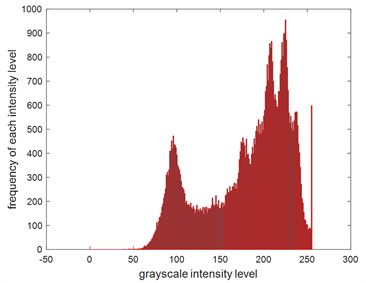(a)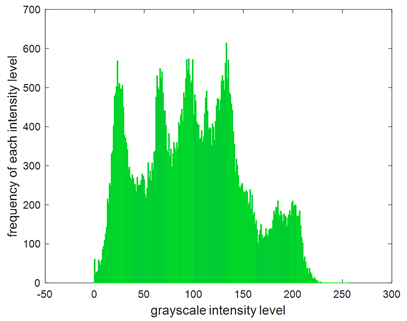(b)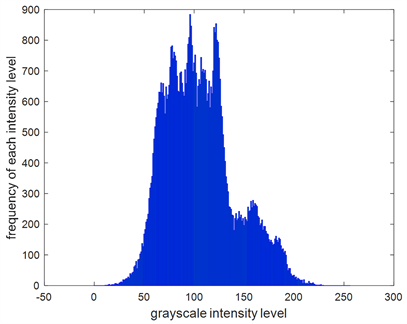(c)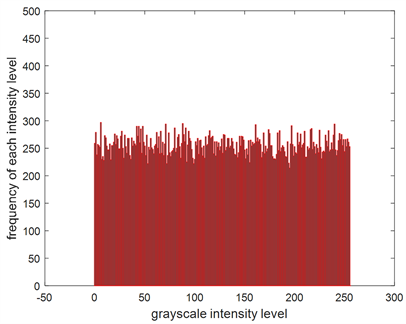(d)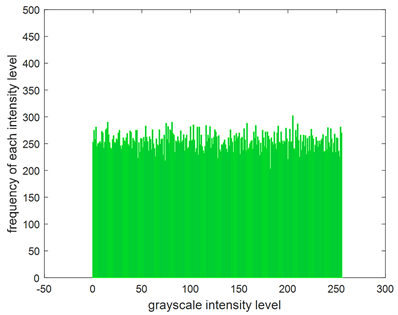(e)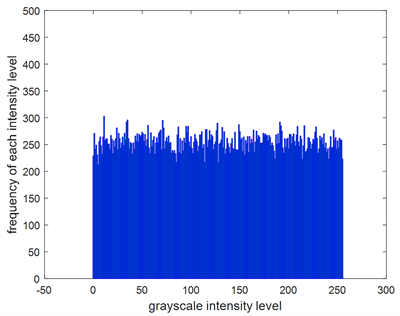(f)

Figure 2. Histogram analysis: (a) Lena plaintext R component histogram; (b) Lena plaintext G component histogram; (c) Lena plaintext B component histogram; (d) Lena ciphertext R component histogram; (e) Lena ciphertext G component histogram; (f) Lena ciphertext B component histogram

$\begin{array}{l}{r}_{xy}=\frac{cov\left(x,y\right)}{\sqrt{D\left(x\right)}\sqrt{D\left(y\right)}},\text{\hspace{0.17em}}cov\left(x,y\right)=\frac{1}{T}{\sum }_{i=1}^{T}\left({x}_{i}-E\left(x\right)\right)\left({y}_{i}-E\left(y\right)\right),\\ D\left(x\right)=\frac{1}{T}{\sum }_{i=1}^{T}{\left({x}_{i}-E\left(x\right)\right)}^{2},\text{\hspace{0.17em}}E\left(x\right)=\frac{1}{T}{\sum }_{i=1}^{T}{x}_{i}.\end{array}$ (21)Table 3. Correlation coefficient between adjacent pixels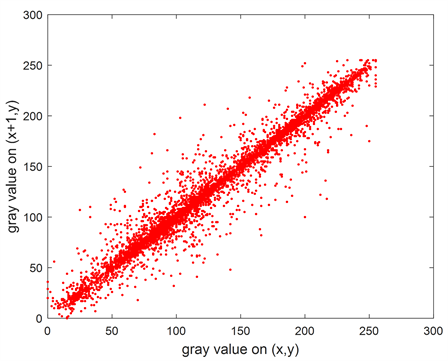(a)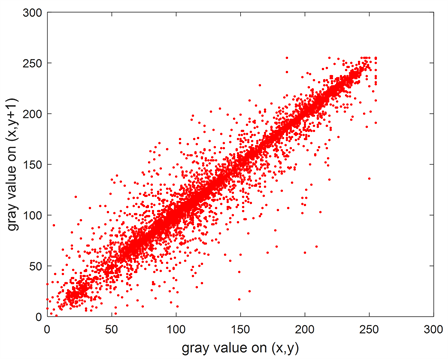(b)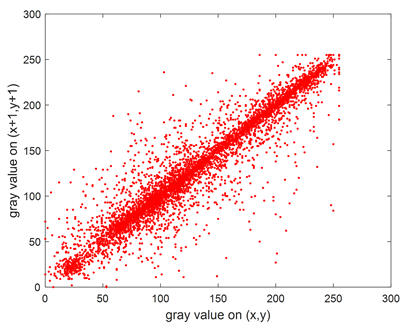(c)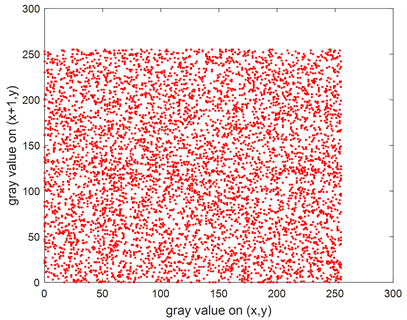(d)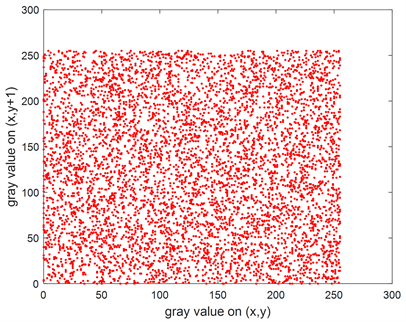(e)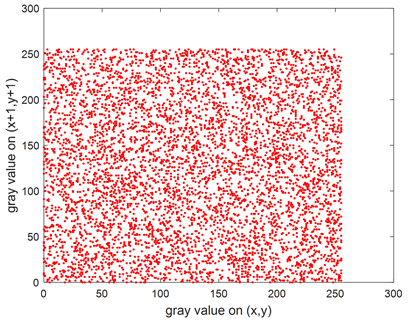(f)

Figure 3. Correlation distribution of adjacent pixels of Lena plaintext and its ciphertext image: (a) Plaintext horizontal direction; (b) Plaintext vertical direction; (c) Plaintext diagonal direction; (d) Ciphertext horizontal direction; (e) Ciphertext vertical direction; (f) Ciphertext diagonal direction

5.4. 抗差分攻击分析

5.5. 信息熵分析

$H\left(s\right)=-{\sum }_{i=1}^{L}P\left({s}_{i}\right)\mathrm{log}P\left({s}_{i}\right)$ (22)

6. 总结

NOTES

*通讯作者。

  Stinson, D.R. (1995) Cryptography: Theory and Practice. CRC Press, Boca Raton.  Fridrich, J. (1998) Symmetric Ciphers Based on Two-Dimensional Chaotic Maps. International Journal of Bifurcation and Chaos, 8, 1259-1284. https://doi.org/10.1142/S021812749800098X  Ye, R. (2011) A Novel Chaos-Based Image Encryption Scheme with an Efficient Permutation-Diffusion Mechanism. Optics Communications, 284, 5290-5298. https://doi.org/10.1016/j.optcom.2011.07.070  Tang, Z., Song, J., Zhang, X. and Sun, R. (2016) Multiple-Image Encryption with Bit-Plane Decomposition and Chaotic Maps. Optics and Lasers in Engineering, 80, 1-11. https://doi.org/10.1016/j.optlaseng.2015.12.004  Ye, R. (2014) A Novel Image Encryption Scheme Based on Generalized Multi-Sawtooth Maps. Fundamenta Informaticae, 133, 87-104. https://doi.org/10.3233/FI-2014-1063  Ding, C., Pei, D. and Salomaa, A. (1996) Chinese Remainder Theorem (Applications in Computing, Coding, Cryptography). World Scientific, Singapore. https://doi.org/10.1142/3254  Zhu, H., Zhao, C. and Zhang, X. (2013) A Novel Image Encryption-Compression Scheme Usinghyper-Chaos and Chinese Remainder Theorem. Signal Process. Image Communication, 28, 670-680. https://doi.org/10.1016/j.image.2013.02.004  Brindhaa, M. and Ammasai Goundenb, N. (2016) A Chaos-Based Image Encryption and Lossless Compression Algorithm Using Hash Table and Chinese Remainder Theorem. Applied Soft Computing, 40, 379-390. https://doi.org/10.1016/j.asoc.2015.09.055  Ye, R. and Zhou, W. (2011) An Image Encryption Scheme Based on 2D Tent Map and Coupled Map Lattice. International Journal of Information & Communication Technology Research, 1, 344-348.  Alvarez, G. and Li, S.J. (2006) Some Basic Cryptographic Requirements for Chaos-Based Cryptosystems. International Journal of Bifurcation & Chaos, 16, 2129-2151. https://doi.org/10.1142/S0218127406015970  Wu, Y., Noonan, J.P. and Agaian, S. (2011) NPCR and UACI Randomness Tests for Image Encryption. Journal of Selected Areas in Telecommunications (JSAT), 1, 31-38.  Shannon, C.E. (1949) Communication Theory of Secrecy Systems. Bell System Technical Journal, 28, 656-715. https://doi.org/10.1002/j.1538-7305.1949.tb00928.x  Petrou, Maria. Image Processing: The Fundamentals. Second Edition. Wiley, Hoboken, New Jersey, USA, 2010. https://doi.org/10.1002/9781119994398  Wen, W., Zhang, Y., Su, M., et al. (2017) Differential Attack on a Hyper-Chaos-Based Image Cryptosystem with a Classic Bi-Modular Architecture. Nonlinear Dynamics, 87, 383-390. https://doi.org/10.1007/s11071-016-3049-x